# SQL Query to Convert Datetime to String

• Last Updated : 25 Oct, 2021

In order to convert a DateTime to a string, we can use CONVERT() and CAST() function. These functions are used to converts a value(of any datatype) into a specified datatype.

### CONVERT() Function

Syntax:

Attention reader! Don’t stop learning now. Learn SQL for interviews using SQL Course  by GeeksforGeeks.

`CONVERT(VARCHAR, datetime [,style])`
• VARCHAR – It represent the string type.
• datetime – It can be the expression that evaluates date or datetime value that you want to convert into string.
• style – It specifies the format of the date. It’s value is predefined by the SQL Server. The style parameter is optional.

### CAST() Function

Syntax:

`CAST(EXPRESSION AS DATATYPE(length))`
• EXPRESSION – It represent the value that need to be converted.
• DATATYPE – It is the type of the data to which we want to convert our expression.
• length – It represent the length of the resulting datatype(optional).

In the below example, we will convert the DateTime into a string in different formats.

Step 1: Create a database

Query:

`CREATE DATABASE Product_order;`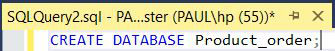Figure: Create DATABASE

Step 2: Create a table

Now, we need to create a table inside our database. For this, we will use CREATE statement.

Query:

```CREATE TABLE orders (prod_id INT,
prod_name VARCHAR(255),
order_date DATE,
PRIMARY KEY(prod_id));```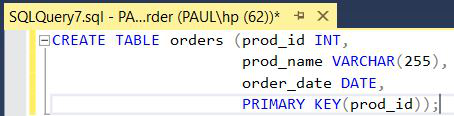Figure: Create a table orders

Step 3: Insert data into a table

In this step, we will insert data inside our orders table. For inserting data we will use an INSERT statement.

Query:

```INSERT INTO orders VALUES (101, 'iPhone', '2020-07-20'),
(103, 'iWatch', '2019-03-15'),
(104, 'iMac', '2016-05-13');```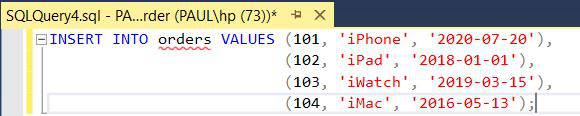Figure: Insert data into the order table

Step 4: In order to verify the contents of the table, we will be using the SELECT statement.

`SELECT * FROM orders;`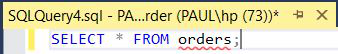Figure: Select statement query

Output: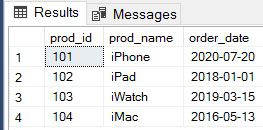Figure: Order table

Step 5: Using CONVERT() function

Query :

```/*Declaring DATETIME as dt*/
DECLARE @dt DATETIME = (SELECT order_date
FROM orders WHERE prod_id = 101);
/*SELECT statement is used to print the s1 message*/
SELECT
CONVERT(VARCHAR(20),@dt,0) s1;```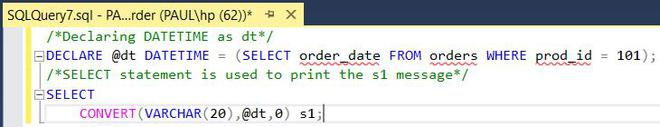Figure: CONVERT() function query

Output: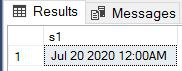Figure: Output

Query :

In this, we are changing the style parameter to 1. Similarly, you can use different style parameter values from the above table.

```/*Declaring DATETIME as dt*/
DECLARE @dt DATETIME = (SELECT order_date
FROM orders WHERE prod_id = 103);
/*SELECT statement is used to print the s1 message*/
SELECT
CONVERT(VARCHAR(20),@dt,1) s1;```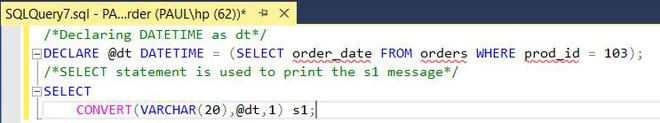Figure: CONVERT() function query

Output :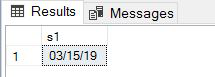Figure: Output

Step 6: Using CAST() function

Query:

```/*Declaring DATETIME as dt*/
DECLARE @dt DATETIME = (SELECT order_date
FROM orders WHERE prod_id = 102);
/*SELECT statement is used to print the s1 message*/
SELECT
CAST(@dt AS DATETIME) s1;```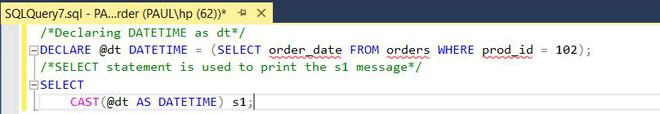Figure: CAST() function query

Output: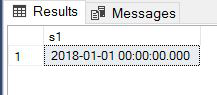Figure: Output

My Personal Notes arrow_drop_up